• a--) { while(a*a+b*b*R)b++; b--; count+=a*(b-tb); tb=b; } System.out.println(count*4); //答案 3137548 } } 3.标题：复数幂 设i为虚数单位。对于任意正整数n，(2+3i)^n 的实部和虚部都是整数。 求 (2+3i)^...
1：第几天2000年的1月1日，是那一年的第1天。那么，2000年的5月4日，是那一年的第几天？注意：需要提交的是一个整数，不要填写任何多余内容。用excel算，答案125.2.标题：方格计数如图p1.png所示，在二维平面上有无数个1x1的小方格。我们以某个小方格的一个顶点为圆心画一个半径为1000的圆。你能计算出这个圆里有多少个完整的小方格吗？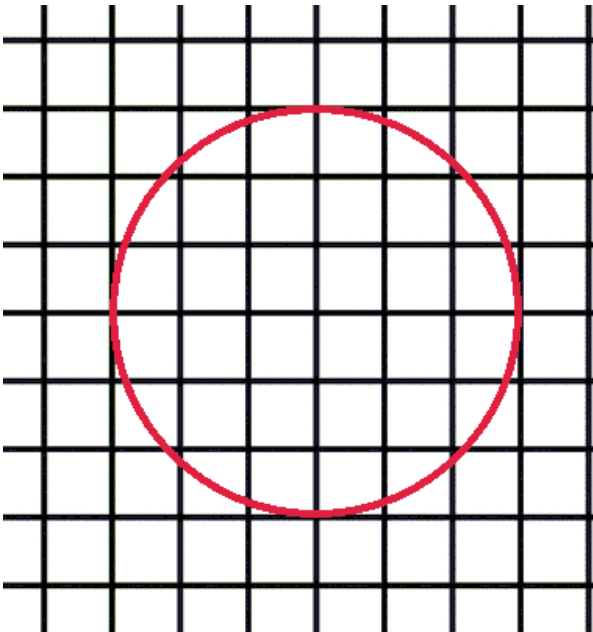注意：需要提交的是一个整数，不要填写任何多余内容。public class Main {public static void main(String[] args) {int R=1000;int a=1000,b=0,tb=b,count=0;for(a=1000;a>=0;a--) {while(a*a+b*b<=R*R)b++;b--;count+=a*(b-tb);tb=b;}System.out.println(count*4);    //答案 3137548}}3.标题：复数幂设i为虚数单位。对于任意正整数n，(2+3i)^n 的实部和虚部都是整数。求 (2+3i)^123456 等于多少？ 即(2+3i)的123456次幂，这个数字很大，要求精确表示。答案写成 "实部±虚部i" 的形式，实部和虚部都是整数(不能用科学计数法表示)，中间任何地方都不加空格，实部为正时前面不加正号。(2+3i)^2 写成: -5+12i，(2+3i)^5 的写成: 122-597i注意：需要提交的是一个很庞大的复数，不要填写任何多余内容。注意点：1.直接在控制台输出的话，因为太大了，不会显示完整数字结果，结果如图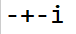，其实，粘贴到记事本就可以看到完整的。2.或者直接用FileWrite()写入文本。import java.io.FileWriter;import java.io.IOException;import java.math.BigInteger;import java.util.Scanner;public class Main {public static Scanner cin = new Scanner(System.in);public static void main(String[] args) throws IOException {//求 (2+3i)^123456 等于多少？//存答案BigInteger a = new BigInteger("2");BigInteger b = new BigInteger("3");//乘数BigInteger c = new BigInteger("2");BigInteger d = new BigInteger("3");//注意我是从第二个开始的：for(int i = 1; i < 123456; i++) {BigInteger x = a.multiply(c).subtract(b.multiply(d));BigInteger y = a.multiply(d).add(b.multiply(c));a = x;b = y;}//System.out.println(a + "+" + d + "i");//写文件FileWriter file = new FileWriter("ans.txt");//需要判断虚部的正负号，如果是负的就不需要"+"if(b.compareTo(new BigInteger("0")) < 0) {System.out.println("b < 0");file.write(a + "" + d + "i");}else if (b.compareTo(new BigInteger("0")) > 0){System.out.println("b > 0");file.write(a + "+" + d + "i");}else {System.out.println("b = 0");file.write(a + "");}file.close();}}4.标题：测试次数x星球的居民脾气不太好，但好在他们生气的时候唯一的异常举动是：摔手机。各大厂商也就纷纷推出各种耐摔型手机。x星球的质监局规定了手机必须经过耐摔测试，并且评定出一个耐摔指数来，之后才允许上市流通。x星球有很多高耸入云的高塔，刚好可以用来做耐摔测试。塔的每一层高度都是一样的，与地球上稍有不同的是，他们的第一层不是地面，而是相当于我们的2楼。如果手机从第7层扔下去没摔坏，但第8层摔坏了，则手机耐摔指数=7。特别地，如果手机从第1层扔下去就坏了，则耐摔指数=0。如果到了塔的最高层第n层扔没摔坏，则耐摔指数=n为了减少测试次数，从每个厂家抽样3部手机参加测试。某次测试的塔高为1000层，如果我们总是采用最佳策略，在最坏的运气下最多需要测试多少次才能确定手机的耐摔指数呢？请填写这个最多测试次数。注意：需要填写的是一个整数，不要填写任何多余内容。分案一：数学推导：注：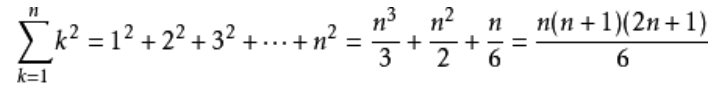方案二：网上说：这道题其实是需要用动态规划或者记忆化搜索去解的，dp[x][y]表示当前还剩x楼不确定，还有y部手机没爆。尚未看懂！！！！！！！！！！#include#define Max(a,b) (a>b?a:b)#define Min(a,b) (aint dp;int main(int argc, char* argv[]){int n,m;scanf("%d%d",&n,&m);for (int i=1;i<=n;i++){dp[i]=i;}for (int cnt=2;cnt<=m;cnt++){for (int ind=1;ind<=n;ind++){dp[ind][cnt]=1+dp[ind-1][cnt];for (int k=2;k<=ind;k++)dp[ind][cnt]=Min(dp[ind][cnt],1+Max(dp[k-1][cnt-1],dp[ind-k][cnt]));}}printf("%d\n",dp[n][m]);return 0;}第五题：标题：快速排序以下代码可以从数组a[]中找出第k小的元素。它使用了类似快速排序中的分治算法，期望时间复杂度是O(N)的。请仔细阅读分析源码，填写划线部分缺失的内容。import java.util.Random;public class Main{public static int quickSelect(int a[], int l, int r, int k) {Random rand = new Random();int p = rand.nextInt(r - l + 1) + l;int x = a[p];int tmp = a[p]; a[p] = a[r]; a[r] = tmp;int i = l, j = r;while(i < j) {while(i < j && a[i] < x) i++;if(i < j) {a[j] = a[i];j--;}while(i < j && a[j] > x) j--;if(i < j) {a[i] = a[j];i++;}}a[i] = x;p = i;if(i - l + 1 == k) return a[i];if(i - l + 1 < k) return quickSelect( _________________________________ ); //填空else return quickSelect(a, l, i - 1, k);}public static void main(String args[]) {int [] a = {1, 4, 2, 8, 5, 7};System.out.println(quickSelect(a, 0, 5, 4));}}注意：只提交划线部分缺少的代码，不要抄写任何已经存在的代码或符号。我当时的答案是：a, i + 1, r, k - i  ， 当时跑了下，结果正确就没管了，实际上如果我多测计组就会发现是错的。答案应该是： a, i + 1, r, k - i + l - 1；对于那个传过去的 第几大问题，不能直接以为 k - i 就行了，因为 k 是新的，新对于一截数组的元素个数的第几大，然而 那个下标 i  依然是整个数组的 i，所以 k 有种情况会小于 i ，故应该在数组中找到真实的 第几大，再去减掉 i, 故因为是 k + l  - i + 1-----------------------------------编程题-------------------------------------------第六题给定三个整数数组 A = [A1, A2, ... AN], B = [B1, B2, ... BN], C = [C1, C2,... CN]， 请你统计有多少个三元组(i, j, k) 满足：1 <= i, j, k <= NAi < Bj < Ck【输入格式】 第一行包含一个整数N。 第二行包含N个整数A1, A2, ... AN。 第三行包含N个整数B1, B2, ... BN。第四行包含N个整数C1, C2, ... CN。对于30%的数据，1 <= N <= 100对于60%的数据，1 <= N <= 1000对于100%的数据，1 <= N <=100000 0 <= Ai, Bi, Ci <= 100000【输出格式】 一个整数表示答案【输入样例】31 1 12 2 23 3 3【输出样例】27import java.util.Arrays;import java.util.Comparator;import java.util.Scanner;public class Main{public static void main(String args[] ) {int n;Scanner cin = new Scanner(System.in);n = cin.nextInt();Integer[] a = new Integer[n];Integer[] b = new Integer[n];Integer[] c = new Integer[n];for(int i = 0; i < n; i++) {a[i] = cin.nextInt();}for(int i = 0; i < n; i++) {b[i] = cin.nextInt();}for(int i = 0; i < n; i++) {c[i] = cin.nextInt();}Comparator cmp = new MyComparator();Arrays.sort(a, cmp);Arrays.sort(b, cmp);Arrays.sort(c, cmp);int[] bc = new int[n]; //存放bj > ck 的个数long[] he = new long[n]; //存放bc的加和long ans = 0;int i, j;for(i = 0; i < n; i++) {for(j = 0; j < n; j++) {if(b[i] >= c[j]) {break;}}bc[i] = j;if(i == 0) {he[i] = j;}else {he[i] = he[i-1] + j;}//System.out.print(bc[i] + ",");}for(i = 0; i < n; i++) {for(j = 0; j < n; j++) {if(a[i] >= b[j]) {break;}}if(j > 0)ans += he[j-1];}System.out.println(ans);}}class MyComparator implements Comparator{@Overridepublic int compare(Integer o1, Integer o2) {return o2 - o1;}}/*31 1 12 1 23 2 312-------31 1 12 2 23 3 327*/第七题标题：螺旋折线如图p1.pgn所示的螺旋折线经过平面上所有整点恰好一次。对于整点(X, Y)，我们定义它到原点的距离dis(X, Y)是从原点到(X, Y)的螺旋折线段的长度。例如dis(0, 1)=3, dis(-2, -1)=9给出整点坐标(X, Y)，你能计算出dis(X, Y)吗？【输入格式】X和Y对于40%的数据，-1000 <= X, Y <= 1000对于70%的数据，-100000 <= X， Y <= 100000对于100%的数据, -1000000000 <= X, Y <= 1000000000【输出格式】输出dis(X, Y)【输入样例】0 1【输出样例】3资源约定：峰值内存消耗(含虚拟机) < 256MCPU消耗  < 1000ms请严格按要求输出，不要画蛇添足地打印类似：“请您输入...” 的多余内容。---------------------思路：记得我去年是分象限讨论的，找了每个对角线的点为基准，然后讨论，细心点也好搞出来，但是比较复杂。今年重新看下，发现有了新思路(刚刚看到有网上有图，直接搞过来吧，自己懒得画了)：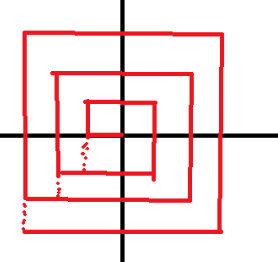就是弄成完整的正方形，这样子就好搞多了，也就是说你可以总结出一个公式了：以(-n, -n)为基准，所以就是最外圈 + 内圈和了。内圈是： 1*8 + 2 * 8 + 3 * 8 + 。。。+ ( max(abs(x), abs(y) )  -  1) * 8外圈就是：if y < x : max(abs(x), abs(y) ) * 8 -  ( abs(x - max(abs(x), abs(y) )  +  abs(y - max(abs(x), abs(y) ) )else :  abs(x - max(abs(x), abs(y) )  +  abs(y - max(abs(x), abs(y) )总数量就是 ： 内圈加外圈之和了。第八题：日志统计小明维护着一个程序员论坛。现在他收集了一份"点赞"日志，日志共有N行。其中每一行的格式是：ts id表示在ts时刻编号id的帖子收到一个"赞"。现在小明想统计有哪些帖子曾经是"热帖"。如果一个帖子曾在任意一个长度为D的时间段内收到不少于K个赞，小明就认为这个帖子曾是"热帖"。具体来说，如果存在某个时刻T满足该帖在[T, T+D)这段时间内(注意是左闭右开区间)收到不少于K个赞，该帖就曾是"热帖"。给定日志，请你帮助小明统计出所有曾是"热帖"的帖子编号。【输入格式】 第一行包含三个整数N、D和K。 以下N行每行一条日志，包含两个整数ts和id。对于50%的数据，1 <= K <= N <= 1000对于100%的数据，1 <= K <= N <= 1000000 <= ts<= 100000 0 <= id <= 100000【输出格式】 按从小到大的顺序输出热帖id。每个id一行。【输入样例】 7 10 20 10 1010 1010 19 1100 3100 3【输出样例】 1 3资源约定： 峰值内存消耗(含虚拟机) < 256M CPU消耗 < 1000ms请严格按要求输出，不要画蛇添足地打印类似：“请您输入...” 的多余内容。所有代码放在同一个源文件中，调试通过后，拷贝提交该源码。 不要使用package语句。不要使用jdk1.7及以上版本的特性。主类的名字必须是：Main，否则按无效代码处理。暴力：先按时间排序，然后两重for判断，用了hashmap统计，set去重统计id后面的代码用了尺取法。import java.util.Arrays;import java.util.Comparator;import java.util.HashMap;import java.util.HashSet;import java.util.Iterator;import java.util.Scanner;public class Main{public static void main(String args[] ) {int n, d, k;Scanner cin = new Scanner(System.in);n = cin.nextInt();d = cin.nextInt();k = cin.nextInt();zan[] a = new zan[n ];for(int i = 0; i < n; i++) {a[i] = new zan(cin.nextInt(), cin.nextInt());}Arrays.sort(a, new Comparator() {public int compare(zan x, zan y) {return x.ts - y.ts;}});//for(zan i : a) {//System.out.println(i.ts);//}//用hashmap统计每个时段内每个内容的点赞次数HashMap map = new HashMap<>();HashSet set = new HashSet<>();for(int i = a.ts; i <= a[n-1].ts; i++) {for(int j = 0; j < n; j++) {if(a[j].ts >= i && a[j].ts < i + d) {if(map.containsKey(a[j].id)) {int tm = map.get(a[j].id) + 1;if(tm >= k && !set.contains(a[j].id)) {set.add(a[j].id);//set.}map.put(a[j].id, tm);}else {map.put(a[j].id, 1);}}}//System.out.println(map.values());map.clear();}//System.out.println(set.size() + ": " + set.toString());//取出所有的set内容，存入ans中Iterator it = set.iterator();int[] ans = new int[set.size()];int ct = 0;while(it.hasNext()) {ans[ct++] = it.next();//System.out.print(it.next() + " ");}Arrays.sort(ans);for(int i = 0; i < ct; i++) {System.out.print(ans[i] + " ");}}}class zan{public int ts;public int id;zan(int t, int i){this.ts = t;this.id = i;}}/*7 10 20 10 1010 1010 19 1100 3100 3*/import java.util.ArrayList;import java.util.Arrays;import java.util.Collections;import java.util.HashSet;import java.util.List;import java.util.Scanner;public class Main{public static int n, d, k;public static List[] list = new ArrayList;public static void main(String args[] ) {Scanner cin = new Scanner(System.in);n = cin.nextInt();d = cin.nextInt();k = cin.nextInt();int Ids = 100005;for(int i = 0; i < Ids; i++) {list[i] = new ArrayList();}HashSet set = new HashSet<>();for(int i = 0; i < n; i++) {int id, ts;ts = cin.nextInt();id = cin.nextInt();list[id].add(ts);set.add(id);}int cnt = 0, ids = set.size();int[] ans = new int[ids];Object[] yid = new Object[ids];//将集合里面的数据取出来yid = set.toArray();for(int i = 0; i < ids; i++) {if(judge((int) yid[i])) {ans[cnt++] = (int)yid[i];//System.out.println(yid[i] + "-----");}}for(int i = 0; i < cnt; i++) {System.out.print(yid[i] + " ");}}public static boolean judge(int id) {int l = 0, r = 0;int len = list[id].size();int sum = 0;if(len < k) {return false;}//对链表排序Collections.sort(list[id]);//尺取法while(l <= r && r < len) {if(r - l + 1 >= k) {if(list[id].get(r) - list[id].get(l) < d) {return true;}else {l++;}}r++;}return false;}}/*7 10 20 10 1010 1010 19 1100 3100 3*/第九题 全球变暖你有一张某海域NxN像素的照片，"."表示海洋、"#"表示陆地，如下所示：........##.....##........##...####....###........其中"上下左右"四个方向上连在一起的一片陆地组成一座岛屿。例如上图就有2座岛屿。由于全球变暖导致了海面上升，科学家预测未来几十年，岛屿边缘一个像素的范围会被海水淹没。具体来说如果一块陆地像素与海洋相邻(上下左右四个相邻像素中有海洋)，它就会被淹没。例如上图中的海域未来会变成如下样子：................................#................请你计算：依照科学家的预测，照片中有多少岛屿会被完全淹没。【输入格式】第一行包含一个整数N。  (1 <= N <= 1000)以下N行N列代表一张海域照片。照片保证第1行、第1列、第N行、第N列的像素都是海洋。【输出格式】一个整数表示答案。【输入样例】7........##.....##........##...####....###........【输出样例】1资源约定：峰值内存消耗(含虚拟机) < 256MCPU消耗  < 1000ms请严格按要求输出，不要画蛇添足地打印类似：“请您输入...” 的多余内容。思路：把'.'当做0，把'#'当做-1(化作整数方便标记连通分量)1.dfs一次，并在dfs的过程中标连通分量(每个岛给一个编号p，p从1开始)2.在1的过程中，把会被淹没的陆地(如果周围存在海)给予特殊编号-23.最后统计非特殊编号个数cnt, 答案就是p-cnt;注意数据的输入，java 我输入还不是很熟啊！！！import java.util.ArrayList;import java.util.Arrays;import java.util.Collections;import java.util.HashSet;import java.util.List;import java.util.Scanner;public class Main{public static int[][] map;public static int[][] visit;public static int dx[] = {1, -1, 0, 0};public static int dy[] = {0, 0, 1, -1};public static int n;public static void main(String args[] ) {Scanner cin = new Scanner(System.in);n = cin.nextInt();//cin.nextLine();  //读掉回车map = new int[n][n];visit = new int[n][n];for(int i = 0; i < n; i++) {String temp = cin.next();//System.out.println("---" + temp + "----");for(int j = 0; j < n; j++) {if(temp.charAt(j) == '.') {map[i][j] = -1; //水}else {map[i][j] = 0;  //土地}visit[i][j] = 0;}}int cnt = 0; //原来总的岛屿数量for(int i = 0; i < n; i++) {for(int j = 0; j < n; j++) {if(map[i][j] == 0) {cnt++;dfs(i, j, cnt);}}}int ans = 0;HashSet set = new HashSet<>();for(int i = 0; i < n; i++) {for(int j = 0; j < n; j++) {if(map[i][j] > 0) {set.add(map[i][j]);}}}System.out.println(cnt - set.size());System.out.println(set.toString());for(int i = 0; i < n; i++) {for(int j = 0; j < n; j++) {System.out.print(map[i][j]);}System.out.println();}}public static void dfs(int x, int y, int bh) {//System.out.println("x + y" + x + " " + y);map[x][y] = bh;visit[x][y] = 1;int flag = 0;for(int i = 0; i < 4; i++) {int xx = x + dx[i];int yy = y + dy[i];if(xx >= 0 && xx < n &&yy >= 0 && yy < n && visit[xx][yy] == 0) {if(map[xx][yy] == -1) { //周边有一个是水，则淹没map[x][y] = -2; //特殊标记，表示已经淹没的，但不能和水一样，否则会导致他相邻的误判}else if(map[xx][yy] == 0){dfs(xx, yy, bh);}}}}}/*样例1：7........##.....##........##...####....###........1样例2：7........##.....####......##...####....###........0*/10.标题：堆的计数我们知道包含 N 个元素的堆可以看成是一棵包含 N 个节点的完全二叉树。每个节点有一个权值。对于小根堆来说，父节点的权值一定小于其子节点的权值。假设 N 个节点的权值分别是 1~N，你能求出一共有多少种不同的小根堆吗？例如对于 N=4 有如下 3 种：1/ \2 3/41/ \3 2/41/ \2 4/3由于数量可能超过整型范围，你只需要输出结果除以 1000000009 的余数。【输入格式】 一个整数 N。对于 40% 的数据，1 <= N <= 1000对于 70% 的数据，1 <= N <= 10000对于 100% 的数据，1 <= N <= 100000【输出格式】 一个整数表示答案。【输入样例】 4【输出样例】 3资源约定： 峰值内存消耗(含虚拟机) < 256M CPU 消耗 < 1000ms请严格按要求输出，不要画蛇添足地打印类似：“请您输入...” 的多余内容。所有代码放在同一个源文件中，调试通过后，拷贝提交该源码。 不要使用 package 语句。不要使用 jdk1.7 及以上版本的特性。主类的名字必须是：Main，否则按无效代码处理。纯暴力深搜代码：import java.util.ArrayList;import java.util.Arrays;import java.util.Collections;import java.util.HashSet;import java.util.List;import java.util.Scanner;public class Main{public static int[] visit = new int;public static int n;public static int ans = 0;public static int[] tree = new int;public static void main(String args[] ) {Scanner cin = new Scanner(System.in);n = cin.nextInt(); // 树上节点总数find(1);System.out.println(ans);}public static void find(int x) {if(x == n + 1) {for(int i = 1; i <= n; i++) {System.out.print(tree[i] + " ");}System.out.println();ans = (ans + 1) % 1000000009;}int st = x / 2;for(int i = tree[st] + 1; i <= n; i++) {if(visit[i] == 0) {visit[i] = 1;tree[x] = i;find(x+1);visit[i] = 0;tree[x] = 0;}}}}
展开全文• B = [B1, B2, ... BN], C = [C1, C2, ... CN]， 请你统计有多少个三元(i, j, k) 满足： 1. 1 , j, k   2. Ai   【输入格式】 第一行包含一个整数N。 第二行包含N个整数A1, A2, ... AN。 第三行包含N个...
1.第几天

2000年的1月1日，是那一年的第1天。

那么，2000年的5月4日，是那一年的第几天？

注意：需要提交的是一个整数，不要填写任何多余内容。

答案：125

2.方格计数

如图p1.png所示，在二维平面上有无数个1x1的小方格。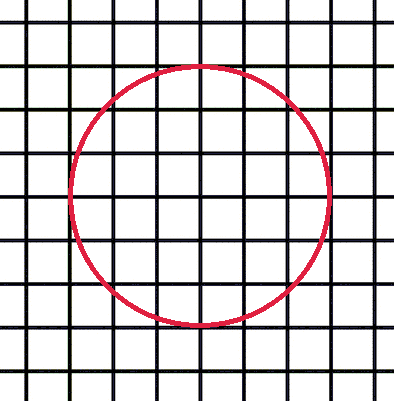我们以某个小方格的一个顶点为圆心画一个半径为1000的圆。
你能计算出这个圆里有多少个完整的小方格吗？

注意：需要提交的是一个整数，不要填写任何多余内容。

呵呵呵呵刚开始算式999*999*4....幸好我18年考的是C++ 虽然填空题我还是只写出了第一题

答案：3137548

import java.util.Scanner;
public class A
{
public static void main(String[] args) {
Scanner sc = new Scanner(System.in);
int cnt = 0 ;
for(int i = 1 ; i <= 1000; i ++)
{
for(int j = 1; j <= 1000; j ++)
{
if(Math.sqrt(i * i + j * j ) <= 1000)
cnt ++;
}
}
System.out.println(cnt * 4);
}
}


3.复数幂

设i为虚数单位。对于任意正整数n，(2+3i)^n 的实部和虚部都是整数。
求 (2+3i)^123456 等于多少？ 即(2+3i)的123456次幂，这个数字很大，要求精确表示。
答案写成 "实部±虚部i" 的形式，实部和虚部都是整数（不能用科学计数法表示），中间任何地方都不加空格，实部为正时前面不加正号。(2+3i)^2 写成: -5+12i，
(2+3i)^5 的写成: 122-597i

注意：需要提交的是一个很庞大的复数，不要填写任何多余内容。

有135kb。。。。。。

4.测试次数
x星球的居民脾气不太好，但好在他们生气的时候唯一的异常举动是：摔手机。
各大厂商也就纷纷推出各种耐摔型手机。x星球的质监局规定了手机必须经过耐摔测试，并且评定出一个耐摔指数来，之后才允许上市流通。
x星球有很多高耸入云的高塔，刚好可以用来做耐摔测试。塔的每一层高度都是一样的，与地球上稍有不同的是，他们的第一层不是地面，而是相当于我们的2楼。
如果手机从第7层扔下去没摔坏，但第8层摔坏了，则手机耐摔指数=7。
特别地，如果手机从第1层扔下去就坏了，则耐摔指数=0。
如果到了塔的最高层第n层扔没摔坏，则耐摔指数=n
为了减少测试次数，从每个厂家抽样3部手机参加测试。
某次测试的塔高为1000层，如果我们总是采用最佳策略，在最坏的运气下最多需要测试多少次才能确定手机的耐摔指数呢？
请填写这个最多测试次数。

注意：需要填写的是一个整数，不要填写任何多余内容。

5.快速排序
以下代码可以从数组a[]中找出第k小的元素。
它使用了类似快速排序中的分治算法，期望时间复杂度是O(N)的。

请仔细阅读分析源码，填写划线部分缺失的内容。

import java.util.Random;
public class Main{
public static int quickSelect(int a[], int l, int r, int k) {
Random rand = new Random();
int p = rand.nextInt(r - l + 1) + l;
int x = a[p];
int tmp = a[p]; a[p] = a[r]; a[r] = tmp;
int i = l, j = r;
while(i < j) {
while(i < j && a[i] < x) i++;
if(i < j) {
a[j] = a[i];
j--;
}
while(i < j && a[j] > x) j--;
if(i < j) {
a[i] = a[j];
i++;
}
}
a[i] = x;
p = i;
if(i - l + 1 == k) return a[i];
if(i - l + 1 < k) return quickSelect( _________________________________ ); //填空
else return quickSelect(a, l, i - 1, k);
}
public static void main(String args[]) {
int [] a = {1, 4, 2, 8, 5, 7};
System.out.println(quickSelect(a, 0, 5, 4));
}
}

注意：只提交划线部分缺少的代码，不要抄写任何已经存在的代码或符号。

答案：a, i+1, r, k-(i-l+1)

6.递增三元组
给定三个整数数组
A = [A1, A2, ... AN],
B = [B1, B2, ... BN],
C = [C1, C2, ... CN]，
请你统计有多少个三元组(i, j, k) 满足：
1. 1 <= i, j, k <= N
2. Ai < Bj < Ck

【输入格式】
第一行包含一个整数N。
第二行包含N个整数A1, A2, ... AN。
第三行包含N个整数B1, B2, ... BN。
第四行包含N个整数C1, C2, ... CN。
对于30%的数据，1 <= N <= 100
对于60%的数据，1 <= N <= 1000
对于100%的数据，1 <= N <= 100000 0 <= Ai, Bi, Ci <= 100000
【输出格式】
一个整数表示答案

【输入样例】
3
1 1 1
2 2 2
3 3 3

【输出样例】
27

资源约定：
峰值内存消耗（含虚拟机） < 256M
CPU消耗  < 1000ms
请严格按要求输出，不要画蛇添足地打印类似：“请您输入...” 的多余内容。

7螺旋折线

如图p1.pgn所示的螺旋折线经过平面上所有整点恰好一次。
对于整点(X, Y)，我们定义它到原点的距离dis(X, Y)是从原点到(X, Y)的螺旋折线段的长度。

例如dis(0, 1)=3, dis(-2, -1)=9

给出整点坐标(X, Y)，你能计算出dis(X, Y)吗？

【输入格式】
X和Y

对于40%的数据，-1000 <= X, Y <= 1000
对于70%的数据，-100000 <= X， Y <= 100000
对于100%的数据, -1000000000 <= X, Y <= 1000000000

【输出格式】
输出dis(X, Y)

【输入样例】
0 1

【输出样例】

3

资源约定：
峰值内存消耗（含虚拟机） < 256M
CPU消耗  < 1000ms
请严格按要求输出，不要画蛇添足地打印类似：“请您输入...” 的多余内容。

8.日志统计

小明维护着一个程序员论坛。现在他收集了一份"点赞"日志，日志共有N行。其中每一行的格式是：
ts id
表示在ts时刻编号id的帖子收到一个"赞"。
现在小明想统计有哪些帖子曾经是"热帖"。如果一个帖子曾在任意一个长度为D的时间段内收到不少于K个赞，小明就认为这个帖子曾是"热帖"。
具体来说，如果存在某个时刻T满足该帖在[T, T+D)这段时间内(注意是左闭右开区间)收到不少于K个赞，该帖就曾是"热帖"。
给定日志，请你帮助小明统计出所有曾是"热帖"的帖子编号。

【输入格式】
第一行包含三个整数N、D和K。
以下N行每行一条日志，包含两个整数ts和id。

对于50%的数据，1 <= K <= N <= 1000
对于100%的数据，1 <= K <= N <= 100000 0 <= ts <= 100000 0 <= id <= 100000

【输出格式】
按从小到大的顺序输出热帖id。每个id一行。

【输入样例】
7 10 2
0 1
0 10
10 10
10 1
9 1
100 3
100 3

【输出样例】
1
3
资源约定：
峰值内存消耗（含虚拟机） < 256M
CPU消耗  < 1000ms
请严格按要求输出，不要画蛇添足地打印类似：“请您输入...” 的多余内容。

9.全球变暖

你有一张某海域NxN像素的照片，"."表示海洋、"#"表示陆地，如下所示：
.......
.##....
.##....
....##.
..####.
...###.
.......
其中"上下左右"四个方向上连在一起的一片陆地组成一座岛屿。例如上图就有2座岛屿。
由于全球变暖导致了海面上升，科学家预测未来几十年，岛屿边缘一个像素的范围会被海水淹没。具体来说如果一块陆地像素与海洋相邻(上下左右四个相邻像素中有海洋)，它就会被淹没。
例如上图中的海域未来会变成如下样子：
.......
.......
.......
.......
....#..
.......
.......
请你计算：依照科学家的预测，照片中有多少岛屿会被完全淹没。
【输入格式】

第一行包含一个整数N。  (1 <= N <= 1000)

以下N行N列代表一张海域照片。
照片保证第1行、第1列、第N行、第N列的像素都是海洋。
【输出格式】
一个整数表示答案。
【输入样例】
7
.......
.##....
.##....
....##.
..####.
...###.
.......
【输出样例】
1
资源约定：
峰值内存消耗（含虚拟机） < 256M
CPU消耗  < 1000ms
请严格按要求输出，不要画蛇添足地打印类似：“请您输入...” 的多余内容。

10.堆的计数

我们知道包含N个元素的堆可以看成是一棵包含N个节点的完全二叉树。
每个节点有一个权值。对于小根堆来说，父节点的权值一定小于其子节点的权值。
假设N个节点的权值分别是1~N，你能求出一共有多少种不同的小根堆吗？
例如对于N=4有如下3种：

1
/ \
2   3
/
4

1
/ \
3   2
/
4

1
/ \
2   4
/
3

由于数量可能超过整型范围，你只需要输出结果除以1000000009的余数。
【输入格式】
一个整数N。
对于40%的数据，1 <= N <= 1000
对于70%的数据，1 <= N <= 10000
对于100%的数据，1 <= N <= 100000
【输出格式】
一个整数表示答案。
【输入样例】
4
【输出样例】
3
资源约定：
峰值内存消耗（含虚拟机） < 256M
CPU消耗  < 1000ms
请严格按要求输出，不要画蛇添足地打印类似：“请您输入...” 的多余内容。

展开全文• import java.util.Calendar; import java.util.Scanner; //2000年的1月1日，是那一年的第1天。 那么，2000年的5月4日，是那一年的第几天？ public class Main { public static void main(String[] args) { ...
第几天
> 2000年的1月1日，是那一年的第1天。 那么，2000年的5月4日，是那一年的第几天？
>

package nunber2;

import java.util.Calendar;
import java.util.Scanner;
//2000年的1月1日，是那一年的第1天。 那么，2000年的5月4日，是那一年的第几天？
public class Main {
public static void main(String[] args) {
Scanner sc=new Scanner(System.in);

Calendar c=Calendar.getInstance();
c.set(2000,01-1,01);//创建一个指定时间的的日期
long start=c.getTimeInMillis();

c.set(2000, 05-1,04);
long finish=c.getTimeInMillis();
System.out.println((finish-start)/1000/60/60/24+1);

}

}


方格计数
如图p1.png所示，在二维平面上有无数个1x1的小方格。
我们以某个小方格的一个顶点为圆心画一个半径为1000的圆。 你能计算出这个圆里有多少个完整的小方格吗？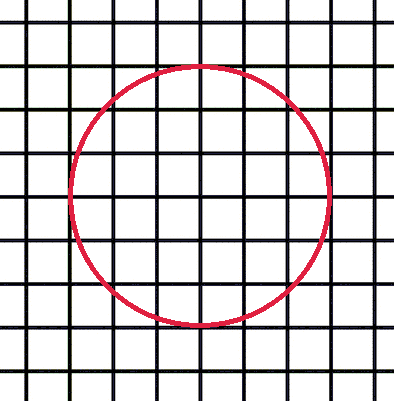package nunber2;

import java.util.Scanner;
//只要这个格子的右上角的坐标与圆心的距离小于圆的半径，就说明这个格子在这个圆内。
public class Main {
public static void main(String[] args) {
Scanner sc=new Scanner(System.in);
//第一象限坐标
int a=1000;//横坐标
int b=1000;//纵坐标

int count=0;
for (int i = 1; i <=1000; i++) {
for (int j = 1; j <=1000; j++) {
int temp=i*i+j*j;
if(temp>1000000)//不完整的格子
count--;
}
}
System.out.println((1000000+count)*4);
}

}


答案：3137548
复数幂
> 设i为虚数单位。对于任意正整数n，(2+3i)^n 的实部和虚部都是整数。
>  求 (2+3i)^123456 等于多少？
> 即(2+3i)的123456次幂，这个数字很大，要求精确表示。 答案写成 "实部±虚部i"
> 的形式，实部和虚部都是整数（不能用科学计数法表示），中间任何地方都不加空格，实部为正时前面不加正号。(2+3i)^2 写成: -5+12i，
> (2+3i)^5 的写成: 122-597i
>
> 注意：需要提交的是一个很庞大的复数，不要填写任何多余内容。

package nunber2;

import java.util.Scanner;

public class Main {
public static void main(String[] args) {
Scanner sc=new Scanner(System.in);
int a=2;//实部
int b=3;//虚部
//刚好是偶数
for (int i = 1; i <123456; i++) {
int temp=a;//避免a的值的改变影响了后面b的值
a=a*2-b*3;
b=2*b+3*temp;
}
System.out.println(a);
System.out.println(b);
}

}


答案：13483137+1100011648i
测试次数
> x星球的居民脾气不太好，但好在他们生气的时候唯一的异常举动是：摔手机。
各大厂商也就纷纷推出各种耐摔型手机。x星球的质监局规定了手机必须经过耐摔测试，并且评定出一个耐摔指数来，之后才允许上市流通。
x星球有很多高耸入云的高塔，刚好可以用来做耐摔测试。塔的每一层高度都是一样的，与地球上稍有不同的是，他们的第一层不是地面，而是相当于我们的2楼。
如果手机从第7层扔下去没摔坏，但第8层摔坏了，则手机耐摔指数=7。 特别地，如果手机从第1层扔下去就坏了，则耐摔指数=0。
如果到了塔的最高层第n层扔没摔坏，则耐摔指数=n
为了减少测试次数，从每个厂家抽样3部手机参加测试。
某次测试的塔高为1000层，如果我们总是采用最佳策略，在最坏的运气下最多需要测试多少次才能确定手机的耐摔指数呢？
请填写这个最多测试次数。
注意：需要填写的是一个整数，不要填写任何多余内容。

package nunber2;

import java.util.Scanner;
public class Main {
public static void main(String[] args) {
Scanner sc=new Scanner(System.in);

System.out.println(solution(1,1000,1000,0));
}
//二分法
public static int solution(int start,int end,int num,int count) {
int index=(start+end)/2;
if(num>index)
return solution(index+1,end,num,count+1);
else if(num==index)
return count+1;//最后一层也要测试一次，不然谁知道最后一层就破了呢
else
return solution(start,index-1,num,count+1);
}

}


快速排序
> 以下代码可以从数组a[]中找出第k小的元素。
它使用了类似快速排序中的分治算法，期望时间复杂度是O(N)的。
请仔细阅读分析源码，填写划线部分缺失的内容。
注意：只提交划线部分缺少的代码，不要抄写任何已经存在的代码或符号。

public class Main{
public static int quickSelect(int a[], int l, int r, int k) {
Random rand = new Random();
int p = rand.nextInt(r - l + 1) + l;
int x = a[p];
int tmp = a[p]; a[p] = a[r]; a[r] = tmp;
int i = l, j = r;
while(i < j) {
while(i < j && a[i] < x) i++;
if(i < j) {
a[j] = a[i];
j--;
}
while(i < j && a[j] > x) j--;
if(i < j) {
a[i] = a[j];
i++;
}
}
a[i] = x;
p = i;
if(i - l + 1 == k) return a[i];
if(i - l + 1 < k) return quickSelect( _________________________________ ); //填空
else return quickSelect(a, l, i - 1, k);
}
public static void main(String args[]) {
int [] a = {1, 4, 2, 8, 5, 7};
System.out.println(quickSelect(a, 0, 5, 4));
}
}

答案：a,i+1,r,k-(i-l+1)
递增三元组
> 给定三个整数数组
A = [A1, A2, ... AN],
B = [B1, B2, ... BN],
C = [C1, C2, ... CN]，
请你统计有多少个三元组(i, j, k) 满足：
>1、 1 <= i, j, k <= N
> 2、 Ai < Bj < Ck
> 【输入格式】
> 第一行包含一个整数N。
> 第二行包含N个整数A1, A2, ... AN。
> 第三行包含N个整数B1, B2, ... BN。
> 第四行包含N个整数C1, C2, ... CN。
> 对于30%的数据，1 <= N <=100
> 对于60%的数据，1 <= N <= 1000
> 对于100%的数据，1 <= N <= 100000 , 0 <= Ai, Bi, Ci <= 100000
> 【输出格式】 一个整数表示答案
> 【输入样例】
>  3
> 1 1 1
> 2 2 2
> 3 3 3
> 【输出样例】 27
> 资源约定： 峰值内存消耗（含虚拟机） < 256M CPU消耗  < 1000ms
>
> 请严格按要求输出，不要画蛇添足地打印类似：“请您输入...” 的多余内容。
所有代码放在同一个源文件中，调试通过后，拷贝提交该源码。
不要使用package语句。不要使用jdk1.7及以上版本的特性。
主类的名字必须是：Main，否则按无效代码处理。

package nunber2;

import java.util.Scanner;
public class Main{
public static void main(String[] args) {
Scanner sc=new Scanner(System.in);
int n=sc.nextInt();
int[] a=new int[n];
int[] b=new int[n];
int[] c=new int[n];

for (int i = 0; i < a.length; i++) {
a[i]=sc.nextInt();
}
for (int i = 0; i < b.length; i++) {
b[i]=sc.nextInt();
}
for (int i = 0; i < c.length; i++) {
c[i]=sc.nextInt();
}

System.out.println(solution(a,b,c,n,0));

}
public static int solution(int[] a,int[] b,int[] c,int n,int count) {
for (int i = 0; i < a.length; i++) {
if(a[i]>n||a[i]<=0)
continue;
for (int j = 0; j < b.length; j++) {
if(b[j]>n||b[j]<=0)
continue;
if(a[i]>=b[j])
continue;
for (int k = 0; k < c.length; k++) {
if(c[k]>n||c[k]<=0)
continue;
if(b[j]>=c[k])
continue;
else
count++;
}
}
}
return count;
}

}


螺旋折线

如图p1.pgn所示的螺旋折线经过平面上所有整点恰好一次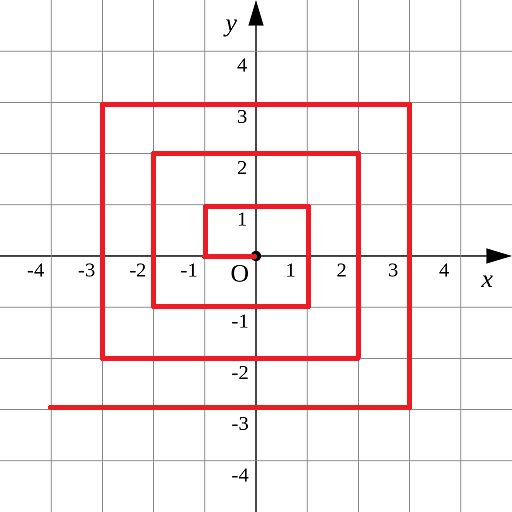>
> 对于整点(X, Y)，我们定义它到原点的距离dis(X, Y)是从原点到(X, Y)的螺旋折线段的长度。
例如dis(0, 1)=3, dis(-2, -1)=9
给出整点坐标(X, Y)，你能计算出dis(X, Y)吗？
【输入格式】
X和Y
对于40%的数据，-1000 <= X, Y <= 1000
对于70%的数据，-100000 <= X， Y <= 100000
对于100%的数据, -1000000000 <= X, Y <= 1000000000
【输出格式】
输出dis(X, Y)
【输入样例】
0 1
【输出样例】
3
资源约定：
峰值内存消耗（含虚拟机） < 256M
CPU消耗  < 1000ms
请严格按要求输出，不要画蛇添足地打印类似：“请您输入...” 的多余内容。
所有代码放在同一个源文件中，调试通过后，拷贝提交该源码。
不要使用package语句。不要使用jdk1.7及以上版本的特性。
主类的名字必须是：Main，否则按无效代码处理。

题解：将每个外围的第一根折线顺时针旋转90°（不影响结果），可以看出是由一个一个正方形构成的图像，所以只需算出所给点内部包围的所有正方形周长ans1和所给点在最外层的折线长度ans2即可；
(x,y)点所在的一个正方形的边长的一半为 a=max(abs(x),abs(y))
ans1=8×(1+2+3+…+a-1)=4a(a-1)

当x>=0时：
若y>=0,ans2=3a+x+a-y=4a+x-y;
若y<0,ans2=5a-y+a-x=6a-x-y;

当x<0：
当y>=0,ans2=2a+x+y
当y<0,ans2=8a-x+y

package nunber2;

import java.util.Scanner;
public class Main{
public static void main(String[] args) {
Scanner sc=new Scanner(System.in);
int x=sc.nextInt();
int y=sc.nextInt();

int a=Math.max(Math.abs(x), Math.abs(y));
int ans1=4*a*(a-1);
int ans2=0;
if(x>=0) {
if(y>=0)
ans2=4*a+x-y;
else
ans2=6*a-x-y;
}else {
if(y>=0)
ans2=2*a+x+y;
else
ans2=8*a-x+y;
}
System.out.println(ans1+ans2);

}
}


日志统计
> 小明维护着一个程序员论坛。现在他收集了一份"点赞"日志，日志共有N行。其中**每一行的格式是：
ts id**
**表示在ts时刻编号id的帖子收到一个"赞"。**
现在小明想统计有哪些帖子曾经是"热帖"。如果一个帖子曾在任意一个长度为D的时间段内收到不少于K个赞，小明就认为这个帖子曾是"热帖"。
具体来说，如果存在某个时刻T满足该帖在[T, T+D)这段时间内(注意是左闭右开区间)收到不少于K个赞，该帖就曾是"热帖"。
给定日志，请你帮助小明统计出所有曾是"热帖"的帖子编号。
【输入格式】
第一行包含三个整数N、D和K。
以下N行每行一条日志，包含两个整数ts和id。
对于50%的数据，1 <= K <= N <= 1000
对于100%的数据，1 <= K <= N <= 100000 0 <= ts <= 100000 0 <= id <= 100000
【输出格式】
按从小到大的顺序输出热帖id。每个id一行。
【输入样例】
7 10 2
0 1
0 10
10 10
10 1
9 1
100 3
100 3
【输出样例】
1
3
资源约定：
峰值内存消耗（含虚拟机） < 256M
CPU消耗  < 1000ms
请严格按要求输出，不要画蛇添足地打印类似：“请您输入...” 的多余内容。
所有代码放在同一个源文件中，调试通过后，拷贝提交该源码。
不要使用package语句。不要使用jdk1.7及以上版本的特性。
主类的名字必须是：Main，否则按无效代码处理。

package nunber2;

import java.util.ArrayList;
import java.util.Arrays;
import java.util.List;
import java.util.Scanner;

public class Main{
public static void main(String[] args) {
Scanner sc=new Scanner(System.in);
int n=sc.nextInt();
int d=sc.nextInt();
int k=sc.nextInt();

List<Integer> id=new ArrayList<Integer>();//id集合
int[][] ts=new int[n][n];
for (int i = 0; i < ts.length; i++) {
Arrays.fill(ts[i], -1);//每个一维数组都赋值-1，不能是二维数组
}
int[] res=new int[n];
Arrays.fill(res, -1);
int index=0;//res的坐标表示

for (int i = 0; i <n; i++) {
int a=sc.nextInt();
int b=sc.nextInt();

if(!id.contains(b))
if(id.contains(b)) {
for (int j = 0; j < ts[id.indexOf(b)].length; j++) {
if(ts[id.indexOf(b)][j]==-1) {
ts[id.indexOf(b)][j]=a;
break;
}
}
}//
}

int balance=1;
for (int i = 0; i < ts.length; i++) {
Arrays.sort(ts[i]);
for (int j = 0; j < ts[i].length; j++) {
if(ts[i][j]==-1)
continue;
for (int j2 =j+1; j2 < ts[i].length; j2++) {
int N=ts[i][j2]-ts[i][j];
if((N)<d)
balance++;
}
if(balance>=k) {
res[index++]=id.get(i);
balance=1;
break;
}
balance=1;
}
}

Arrays.sort(res);
for (int i = 0; i < res.length; i++) {
if(res[i]==-1)
continue;
else
System.out.println(res[i]);
}

}
}


全球变暖

你有一张某海域NxN像素的照片，".“表示海洋、”#"表示陆地，如下所示：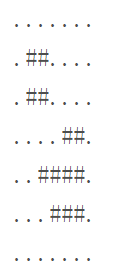其中"上下左右"四个方向上连在一起的一片陆地组成一座岛屿。例如上图就有2座岛屿。
由于全球变暖导致了海面上升，科学家预测未来几十年，岛屿边缘一个像素的范围会被海水淹没。具体来说如果一块陆地像素与海洋相邻(上下左右四个相邻像素中有海洋)，它就会被淹没。
例如上图中的海域未来会变成如下样子：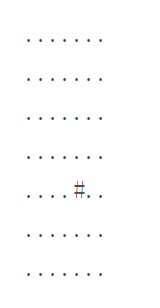请你计算：依照科学家的预测，照片中有多少岛屿会被完全淹没。
【输入格式】 第一行包含一个整数N。  (1 <= N <= 1000)   以下N行N列代表一张海域照片。
【输出格式】
一个整数表示答案。
照片保证第1行、第1列、第N行、第N列的像素都是海洋。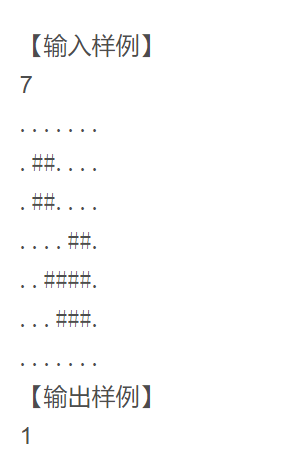package nunber2;

import java.util.Scanner;

public class Main{
public static void main(String[] args) {
Scanner sc=new Scanner(System.in);
int n=sc.nextInt();
char[][] c=new char[n][n];
int count=0;
for (int i = 0; i < c.length; i++) {
c[i]=sc.next().toCharArray();
}

for (int i = 0; i < c.length; i++) {
for (int j = 0; j < c[i].length; j++) {
if(c[i][j]=='#') {//是陆地
if(ifTrue(c, i, j))
continue;
else
count++;
}
}
}
System.out.println(count);

}
//判断是否会沉
public static boolean ifTrue(char[][] c,int i,int j) {
if(c[i-1][j]=='.')//左边
return true;
else if(c[i][j+1]=='.')//上边
return true;
else if(c[i+1][j]=='.')//右边
return true;
else if(c[i][j-1]=='.')//下边
return true;
else
return false;
}
}




展开全文• ## 2018年第九届蓝桥杯B组JAVA题解

千次阅读 多人点赞 2018-04-27 17:45:54
2018年第九届蓝桥杯B组JAVA题解-----------------------------------填空题-------------------------------------------第一题：标题：第几天2000年的1月1日，是那一年的第1天。那么，2000年的5月4日，是那一年的第...


2018年第九届蓝桥杯B组JAVA题解

-----------------------------------填空题-------------------------------------------

第一题：
标题：第几天
2000年的1月1日，是那一年的第1天。

那么，2000年的5月4日，是那一年的第几天？

注意：需要提交的是一个整数，不要填写任何多余内容。

答案：125

第二题 ：

标题：方格计数
如图p1.png所示，在二维平面上有无数个1x1的小方格。我们以某个小方格的一个顶点为圆心画一个半径为1000的圆。
你能计算出这个圆里有多少个完整的小方格吗？

注意：需要提交的是一个整数，不要填写任何多余内容。

import java.util.Scanner;

public class 方格计数 {
static int N = 10000; // 方格图的大小, 10000够大了
static int X = 5000, Y = 5000; // 圆心坐标
static double[] l = new double[N];
static double[] r = new double[N];
static int R, ans = 0;

public static void main(String[] args) {
Scanner sc = new Scanner(System.in);
R = sc.nextInt();
for (int i = Y - R; i <= Y + R; i++) {
l[i] = X - Math.sqrt(R * R - (Y - i) * (Y - i));
r[i] = Math.min(N, (X << 1) - l[i]);
if (l[i] < 0) {
l[i] = 0;
}
}
for (int i = Y - R; i <= Y - 1; i++) {
int ll = (int) Math.ceil(l[i]), rr = (int) Math.floor(r[i]);
ans += rr - ll;
}
for (int i = Y; i <= Y + R - 1; i++) {
int ll = (int) Math.ceil(l[i + 1]), rr = (int) Math.floor(r[i + 1]);
ans += rr - ll;
}
System.out.println(ans);
}
}

我的答案： 3137548

第三题：

标题：复数幂
设i为虚数单位。对于任意正整数n，(2+3i)^n 的实部和虚部都是整数。
求 (2+3i)^123456 等于多少？ 即(2+3i)的123456次幂，这个数字很大，要求精确表示。
答案写成 "实部±虚部i" 的形式，实部和虚部都是整数（不能用科学计数法表示），中间任何地方都不加空格，实部为正时前面不加正号。(2+3i)^2 写成: -5+12i，
(2+3i)^5 的写成: 122-597i

注意：需要提交的是一个很庞大的复数，不要填写任何多余内容。

用java大数加快速幂做的，应该是做对了，但是一开始eclipse控制台没显示出来，以为是大数爆了。

代码：

import java.math.BigInteger;

public class 复数幂 {
public static void main(String[] args) {
Complex cmp = new Complex(BigInteger.valueOf(2), BigInteger.valueOf(3));
System.out.println(quickPow(cmp, 123456).toString());
}

private static Complex quickPow(Complex cmp, int n) {
if (n == 0) {
return new Complex(BigInteger.valueOf(1), BigInteger.valueOf(0));
}
Complex temp = quickPow(cmp, n / 2);
temp.multiply(temp);
if (n % 2 != 0) {
temp.multiply(cmp);
}
return temp;
}
}

class Complex {
BigInteger a;  // 实部
BigInteger b;  // 虚部

public Complex(BigInteger a, BigInteger b) {
this.a = a;
this.b = b;
}

public void multiply(Complex cm) {
BigInteger tempa = a.multiply(cm.a).subtract(b.multiply(cm.b));
a = tempa;
b = tempb;
}

@Override
public String toString() {
String aStr = "" + a;
String bStr = b + "i";
if (b.compareTo(BigInteger.valueOf(0)) > 0) {
bStr = "+" + bStr;
} else if (b.compareTo(BigInteger.valueOf(0)) == 0) {
bStr = "";
}
return aStr + bStr;
}
}

eclipse控制台输出的结果是--i，不知道是什么鬼，比赛完了才知道把这个--i是一个超长的字符串，所以直接把eclipse输出的--i粘贴到记事本就可以看到结果了。

第四题：

标题：测试次数
x星球的居民脾气不太好，但好在他们生气的时候唯一的异常举动是：摔手机。
各大厂商也就纷纷推出各种耐摔型手机。x星球的质监局规定了手机必须经过耐摔测试，并且评定出一个耐摔指数来，之后才允许上市流通。
x星球有很多高耸入云的高塔，刚好可以用来做耐摔测试。塔的每一层高度都是一样的，与地球上稍有不同的是，他们的第一层不是地面，而是相当于我们的2楼。
如果手机从第7层扔下去没摔坏，但第8层摔坏了，则手机耐摔指数=7。
特别地，如果手机从第1层扔下去就坏了，则耐摔指数=0。
如果到了塔的最高层第n层扔没摔坏，则耐摔指数=n
为了减少测试次数，从每个厂家抽样3部手机参加测试。
某次测试的塔高为1000层，如果我们总是采用最佳策略，在最坏的运气下最多需要测试多少次才能确定手机的耐摔指数呢？
请填写这个最多测试次数。

注意：需要填写的是一个整数，不要填写任何多余内容。

100层，问最坏情况下最少几次？这个是最坏情况最多几次，我不知道是不是我多想了，比赛的时候二分答案，错了。。。比赛完结合之前的题目又想了一下，递推了一下，算出来19。

我的答案：19

代码：

public class 摔手机 {
public static void main(String[] args) {
System.out.println(DroppingPhone(3, 1000));
}

private static long DroppingPhone(long phone, long floors) {
long times = 1;
while (DroppingMax(phone, times) < floors) {
++times;
}
return times;
}

private static long DroppingMax(long phone, long times) {
if (phone == 1) {
return times;
}

if (phone >= times) {
return (long) Math.pow(2, times) - 1;
}

return DroppingMax(phone, times - 1) + DroppingMax(phone - 1, times - 1) + 1;
}
}

第五题：

标题：快速排序
以下代码可以从数组a[]中找出第k小的元素。
它使用了类似快速排序中的分治算法，期望时间复杂度是O(N)的。

请仔细阅读分析源码，填写划线部分缺失的内容。

import java.util.Random;
public class Main{
public static int quickSelect(int a[], int l, int r, int k) {
Random rand = new Random();
int p = rand.nextInt(r - l + 1) + l;
int x = a[p];
int tmp = a[p]; a[p] = a[r]; a[r] = tmp;
int i = l, j = r;
while(i < j) {
while(i < j && a[i] < x) i++;
if(i < j) {
a[j] = a[i];
j--;
}
while(i < j && a[j] > x) j--;
if(i < j) {
a[i] = a[j];
i++;
}
}
a[i] = x;
p = i;
if(i - l + 1 == k) return a[i];
if(i - l + 1 < k) return quickSelect( _________________________________ ); //填空
else return quickSelect(a, l, i - 1, k);
}
public static void main(String args[]) {
int [] a = {1, 4, 2, 8, 5, 7};
System.out.println(quickSelect(a, 0, 5, 4));
}
}

注意：只提交划线部分缺少的代码，不要抄写任何已经存在的代码或符号。

利用快排的partition思想进行"选择"，是解决这类问题的高效方法，可以把复杂度从O（nlongn）（直接排序求a[k - 1]）降到O（n）（利用快排分组时左边刚好k-1个元素）。注意题目要求是O(n)，这题如果只求运行正确有几种写法，如果不注意直接写partition 的分治可能还是O（nlogn）。

我的答案：a, i + 1, r, k - i + l - 1

-----------------------------------编程题-------------------------------------------

第六题：

标题：递增三元组
给定三个整数数组
A = [A1, A2, ... AN],
B = [B1, B2, ... BN],
C = [C1, C2, ... CN]，
请你统计有多少个三元组(i, j, k) 满足：
1. 1 <= i, j, k <= N
2. Ai < Bj < Ck
【输入格式】
第一行包含一个整数N。
第二行包含N个整数A1, A2, ... AN。
第三行包含N个整数B1, B2, ... BN。
第四行包含N个整数C1, C2, ... CN。
对于30%的数据，1 <= N <= 100
对于60%的数据，1 <= N <= 1000
对于100%的数据，1 <= N <= 100000 0 <= Ai, Bi, Ci <= 100000
【输出格式】
一个整数表示答案
【输入样例】
3
1 1 1
2 2 2
3 3 3
【输出样例】
27
资源约定：
峰值内存消耗（含虚拟机） < 256M
CPU消耗  < 1000ms
请严格按要求输出，不要画蛇添足地打印类似：“请您输入...” 的多余内容。

这道题的话，可以先排序，然后直接三个for循环（两层）：以B数组为标杆，对于B的每一个元素Bi，二分统计A中比Bi小的元素，C中比Bi大的元素，然后相乘，最后把乘积累加起来。

import java.util.Scanner;

class Main
{
public static void main(String args[])
{
int n;
int a[],b[],c[],d[],e[];
long ans=0;
Scanner sc=new Scanner(System.in);
n=sc.nextInt();
a=new int[n];
b=new int[n];
c=new int[n];
d=new int[n];
e=new int[n];
for(int i=0;i<n;i++)
a[i]=sc.nextInt();
for(int i=0;i<n;i++)
b[i]=sc.nextInt();
for(int i=0;i<n;i++)
c[i]=sc.nextInt();
for(int i=0;i<n;i++)
for(int j=0;j<n;j++)
if(a[i]<b[j])
{
d[i]=j+1;break;
}
for(int i=0;i<n;i++)
for(int j=0;j<n;j++)
if(b[i]<c[j])
{
e[i]=j+1;break;
}
for(int i=0;i<n;i++)
if(d[i]!=0)
for(int j=d[i]-1;j<n;j++)
if(e[j]!=0)
ans+=n-e[j]+1;
System.out.println(ans);
}
}

第七题

标题：螺旋折线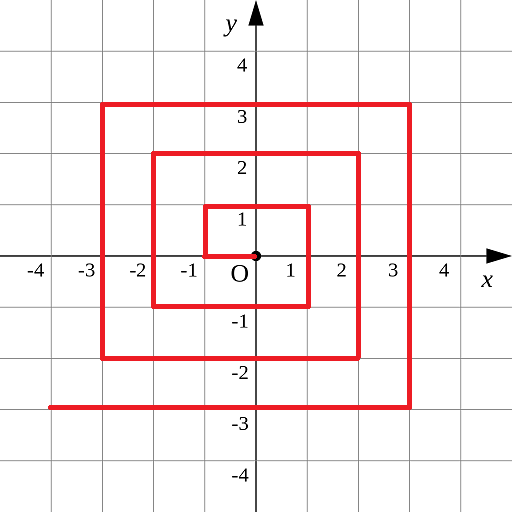如图p1.pgn所示的螺旋折线经过平面上所有整点恰好一次。
对于整点(X, Y)，我们定义它到原点的距离dis(X, Y)是从原点到(X, Y)的螺旋折线段的长度。
例如dis(0, 1)=3, dis(-2, -1)=9
给出整点坐标(X, Y)，你能计算出dis(X, Y)吗？

【输入格式】

X和Y
对于40%的数据，-1000 <= X, Y <= 1000
对于70%的数据，-100000 <= X， Y <= 100000
对于100%的数据, -1000000000 <= X, Y <= 1000000000
【输出格式】
输出dis(X, Y)
【输入样例】
0 1
【输出样例】

3

资源约定：

峰值内存消耗（含虚拟机） < 256M
CPU消耗  < 1000ms
请严格按要求输出，不要画蛇添足地打印类似：“请您输入...” 的多余内容。

这题考察的不是算法，就是思维，方法应该挺多的。方法之一是把螺旋折线转变成一个个的同心正方形，像下面这样，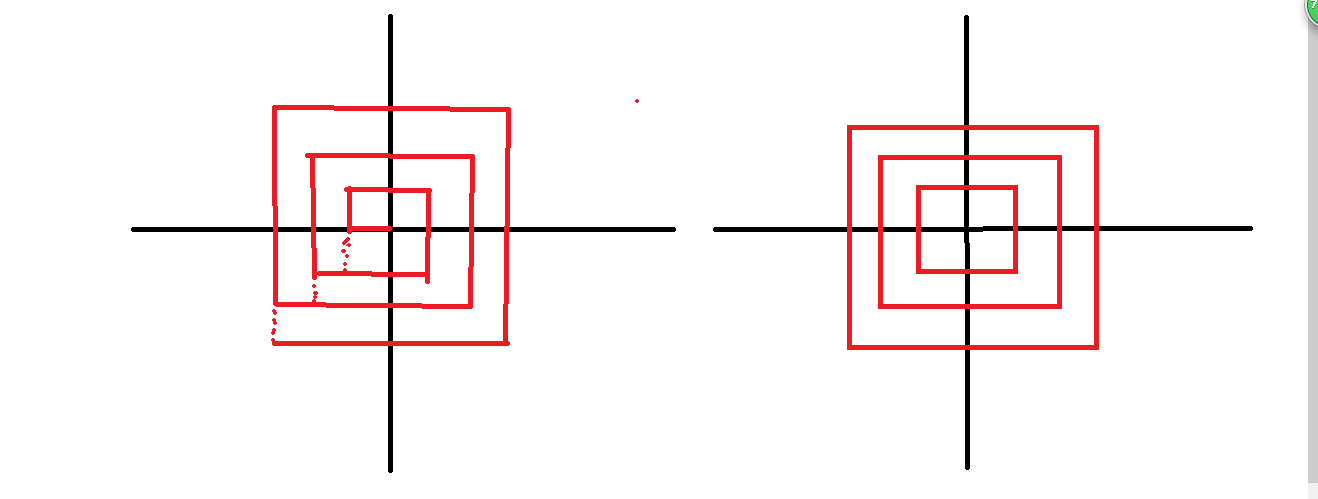注意转化关系，然后就好算多了。

import java.util.Scanner;

class Main
{
public static void main(String args[])
{
int a,b,p,q;
long ans=0;
Scanner sc=new Scanner(System.in);
a=sc.nextInt();
b=sc.nextInt();
if(Math.abs(a)>Math.abs(b))
{
p=a;q=1;
}
else {
p=b;q=2;
}
ans=4*Math.abs(p)*(Math.abs(p)-1);
if(q==1&&a<0)
ans+=b-a;
else if(q==1&&a>=0)
ans+=5*a-b;
else if(q==2&&b<0)
ans=-7*b-a;
else ans+=3*b+a;
System.out.println(ans);
}
}

第八题

标题：日志统计
小明维护着一个程序员论坛。现在他收集了一份"点赞"日志，日志共有N行。其中每一行的格式是：
ts id
表示在ts时刻编号id的帖子收到一个"赞"。
现在小明想统计有哪些帖子曾经是"热帖"。如果一个帖子曾在任意一个长度为D的时间段内收到不少于K个赞，小明就认为这个帖子曾是"热帖"。
具体来说，如果存在某个时刻T满足该帖在[T, T+D)这段时间内(注意是左闭右开区间)收到不少于K个赞，该帖就曾是"热帖"。
给定日志，请你帮助小明统计出所有曾是"热帖"的帖子编号。

【输入格式】

第一行包含三个整数N、D和K。
以下N行每行一条日志，包含两个整数ts和id。
对于50%的数据，1 <= K <= N <= 1000
对于100%的数据，1 <= K <= N <= 100000 0 <= ts <= 100000 0 <= id <= 100000
【输出格式】
按从小到大的顺序输出热帖id。每个id一行。

【输入样例】

7 10 2
0 1
0 10
10 10
10 1
9 1
100 3
100 3
【输出样例】
1
3
资源约定：
峰值内存消耗（含虚拟机） < 256M
CPU消耗  < 1000ms
请严格按要求输出，不要画蛇添足地打印类似：“请您输入...” 的多余内容。

这题用的优先队列加hash（或者直接写一个二维的排序），这题计算点赞量，应该是以每一个点赞时间Ti为为起点，计算[Ti,D)的点赞数（i = 1, 2, 3, ..., n），然后求最大值与K比较。

import java.util.ArrayList;
import java.util.Arrays;
import java.util.Comparator;
import java.util.Scanner;

class Main
{
public static void main(String args[])
{
int n,d,k;
Scanner sc=new Scanner(System.in);
n=sc.nextInt();
d=sc.nextInt();
k=sc.nextInt();
Node arr[]=new Node[n];
for(int i=0;i<n;i++)
arr[i]=new Node(sc.nextInt(),sc.nextInt());
Arrays.sort(arr);
int curid=arr.id;
for(int i=0;i<n;i++)
{
else if(arr[i].id!=curid)
{
curid=arr[i].id;
i=i-1;
}
}

}
}
class Node implements Comparable<Node>
{
int ts,id;
Node(int a,int b)
{
ts=a;
id=b;
}

@Override
public int compareTo(Node o) {
if(id==o.id)
return ts-o.ts;
else
return id-o.id;
}

}



第九题

标题：全球变暖

你有一张某海域NxN像素的照片，"."表示海洋、"#"表示陆地，如下所示：
.......
.##....
.##....
....##.
..####.
...###.
.......
其中"上下左右"四个方向上连在一起的一片陆地组成一座岛屿。例如上图就有2座岛屿。
由于全球变暖导致了海面上升，科学家预测未来几十年，岛屿边缘一个像素的范围会被海水淹没。具体来说如果一块陆地像素与海洋相邻(上下左右四个相邻像素中有海洋)，它就会被淹没。
例如上图中的海域未来会变成如下样子：
.......
.......
.......
.......
....#..
.......
.......
请你计算：依照科学家的预测，照片中有多少岛屿会被完全淹没。
【输入格式】

第一行包含一个整数N。  (1 <= N <= 1000)

以下N行N列代表一张海域照片。

照片保证第1行、第1列、第N行、第N列的像素都是海洋。
【输出格式】
一个整数表示答案。
【输入样例】
7
.......
.##....
.##....
....##.
..####.
...###.
.......
【输出样例】
1
资源约定：
峰值内存消耗（含虚拟机） < 256M
CPU消耗  < 1000ms
请严格按要求输出，不要画蛇添足地打印类似：“请您输入...” 的多余内容。

dfs求联通分量，并且在求联通分量的时候做个标记，判断这个联通分量（岛屿）中是否存在不临海的陆地，存在的话，这样的联通分量（岛屿）才不会完全消失。

import java.util.Scanner;

import org.omg.PortableInterceptor.SYSTEM_EXCEPTION;

class Main
{
static int n,map[][],vis[][];
static boolean dfs(int i,int j)
{
if(i<0||i>=n||j<0||j>=n||vis[i][j]==1) return false;
vis[i][j]=1;
boolean f=false;
if(map[i][j+1]==1&&map[i][j-1]==1&&map[i-1][j]==1&&map[i+1][j]==1)
f=true;
return dfs(i, j+1)||dfs(i, j-1)||dfs(i+1, j)||dfs(i-1, j)||f;
}
public static void main(String args[])
{
int r=0,t=0;
Scanner sc=new Scanner(System.in);
n=sc.nextInt();
map=new int[n][n];
vis=new int[n][n];
for(int i=0;i<n;i++)
{
String s=sc.next();
for(int j=0;j<n;j++)
if(s.charAt(j)!='.')
map[i][j]=1;
else
vis[i][j]=1;
}

for(int i=0;i<n;i++)
for(int j=0;j<n;j++)
if(vis[i][j]!=1)
{

r++;
t+=dfs(i, j)?1:0;

}

System.out.println(r-t);
}
}

第十题

标题：堆的计数
我们知道包含N个元素的堆可以看成是一棵包含N个节点的完全二叉树。
每个节点有一个权值。对于小根堆来说，父节点的权值一定小于其子节点的权值。
假设N个节点的权值分别是1~N，你能求出一共有多少种不同的小根堆吗？
例如对于N=4有如下3种：
1
/ \
2   3
/
4
1
/ \
3   2
/
4
1
/ \
2   4
/
3
由于数量可能超过整型范围，你只需要输出结果除以1000000009的余数。
【输入格式】
一个整数N。
对于40%的数据，1 <= N <= 1000
对于70%的数据，1 <= N <= 10000
对于100%的数据，1 <= N <= 100000
【输出格式】
一个整数表示答案。
【输入样例】
4
【输出样例】
3
资源约定：
峰值内存消耗（含虚拟机） < 256M
CPU消耗  < 1000ms
请严格按要求输出，不要画蛇添足地打印类似：“请您输入...” 的多余内容。

想了一会，写了一个递推式，只验证了4，5两种，后面的没有去验证，不过应该是错了，就不贴了。感觉应该是组合数求和的问题，具体怎么个组合没想清楚，比赛的时候时间有限，没想太多。

本文摘自 https://me.csdn.net/SG_WGL 关于《2018年第九届蓝桥杯B组JAVA题解》


展开全文• 第一题三角形面积已知三角形三个顶点在直角坐标系下的坐标分别为：(2.3, 2.5)(6.4, 3.1)...1 //方法: 推理2 /*3 这是已知三角形3顶点坐标A(x1,y1)，B(x2,y2)，C(x3,y3) ，求三角形ABC的面积的公式4 A为(2.3, 2.5) B...
• public class No_8_2018 {    public static void main(String args[])  {  Scanner s=new Scanner(System.in);  int k=0,n=0,d=0,ksum=0,maxts=0,mints=0,pidno=0;  int[] ts,id,flag,pid;  n=s....
• java.util.ArrayList; 4 import java.util.Arrays; 5 import java.util.HashMap; 6 import java.util.Iterator; 7 import java.util.List; 8 import java.util.Map; 9 import java....
• AN],B = [B1, B2, ... BN],C = [C1, C2, ... CN]，请你统计有多少个三元(i, j, k) 满足：1. 1 <= i, j, k <= N2. Ai < Bj < Ck【输入格式】第一行包含一个整数N。第二行包含N个整数A1, A2, ... AN。...
• 2 3 importjava.util.ArrayList;4 importjava.util.Arrays;5 importjava.util.HashMap;6 importjava.util.Iterator;7 importjava.util.List;8 importjava.util.Map;9 importjava.util.Scanner;10 11 public ...
• 给定三个整数数 A = [A1, A2, … AN], B = [B1, B2, … BN], C = [C1, C2, … CN]， 请你统计有多少个三元(i, j, k) 满足： 1 &amp;amp;amp;lt;= i, j, k &amp;amp;amp;lt;= N Ai &amp;amp;amp;lt; ...全球变暖
• 正在做往年的蓝桥杯赛题，顺手写下自己的思路，新手一名，大佬勿喷~~ 第一题 标题：第几天 2000年的1月1日，是那一年的第1天。 那么，2000年的5月4日，是那一年的第几天？ 注意：需要提交的是一个整数，...
• import java.math.BigInteger; public class Main { public static BigInteger x = BigInteger.valueOf(2); public static BigInteger y = BigInteger.valueOf(3); public static int n = 123456; public ...
• 或者用空间换时间，建立前缀数组，A[i]表示A数组中小于等于i的个数，B[i]表示B数组中小于等于i的个数，以此类推。 7、标题：螺旋折线 如图p1.pgn所示的螺旋折线经过平面上所有整点恰好一次。 对于整点(X, Y)，...
• 蓝桥杯的试题，刚考了，拿了个省二，文件开头有惊喜哦。！！！值得下载。
• AN],B = [B1, B2, ... BN],C = [C1, C2, ... CN]，请你统计有多少个三元(i, j, k) 满足：1. 1 <= i, j, k <= N2. Ai < Bj < Ck【输入格式】第一行包含一个整数N。第二行包含N个整数A1, A2, ... AN。...
• 思路：dfs即可 代码： #include #define mem(a,b) memset(a,b,sizeof(a)) #define mod 1000000007 using namespace std; typedef long long ll; const int maxn = 2e5+5; const double esp = 1e-12; const int ff =...
• 描述 如图p1.png所示，在二维平面上有无数个1x1的小方格。 我们以某个小方格的一个顶点为圆心画一个半径为1000的圆。 你能计算出这个圆里有多少个完整的小方格吗？  ...注意：需要提交的是一个整数，不要填写任何...
• import java.io.FileNotFoundException; import java.io.FileOutputStream; import java.io.PrintStream; import java.math.BigInteger; public class Solution { public static void main(String args[]) throws ...
• 2018-04-01 蓝桥杯个人赛-省赛 Java语言B组全部试题（1-10）
• 2018省赛第九届蓝桥杯真题Java语言B组2018省赛第九届蓝桥杯真题Java语言B组2018省赛第九届蓝桥杯真题Java语言B组2018省赛第九届蓝桥杯真题Java语言B组
• 新鲜出炉的2018蓝桥杯B组真题。先到先得！！！！！！！！！！！！！！！！！！！！！！！！！！！！！！！！！！！！！！！！！！！！！！！！！！！！！！！！！！！！！！！！！！！！！！！！！！！！！！
• 2018省赛B组 题目描述 汉字的字形存在于字库中，即便在今天，16点阵的字库也仍然使用广泛。 16点阵的字库把每个汉字看成是16x16个像素信息。并把这些信息记录在字节中。 一个字节可以存储8位信息，用32个字节就可以...算法
• 然后一分为二： 由图可见，利用勾股定理：矩形的长^2+宽 ^2=斜边 ^2 所以 我们只要保证：a^2+b ^2 ^2 就okay 答案： public class 方格计数 { public static void main(String[] args) { // TODO Auto-generated...
• 顺便说下去年居然拿了国二(蓝桥杯真的水)。。现在回首看来，这些题还蛮简单的，当时怎么也不会的第十题，现在看看也不过是树上的动态规划，先更新第十题(用C++写的，不过java的同学应该看得懂吧) 第十题 堆的计数
• 2018年4月1日愚人节，我第一次参加了有关计算机算法类比赛“蓝桥杯”，这篇算是经验总结和题目回顾，水平有限，有不妥之处欢迎留言批评指正，也可以加QQ891465170交流~下面进入正题：第一题：第几天2000年的1月1日，...
• ## 2018年第九届蓝桥杯B组题解

万次阅读 多人点赞 2018-04-02 12:11:54
题目连接：第九届蓝桥杯试题 C\JAVA 第一题：第几天 答案：125 很简单的数一数就好了，但是我当时可能没带脑子，按2010年算的124天。 第二题：明码 答案：387420489 按着题目把这些数转换成8字节的...
• 解题思路：需要保证第一行、第一列、最后一列和最后一行是’.’... * 2018蓝桥杯Java B组 全球变暖问题： * 条件: .代表海洋像素，#代表像素，相连的一块#代表岛屿，如果陆地像素左右上下某个方向有海洋便会被淹......

# 蓝桥杯b组2018javajava 订阅